# JP2010079252A - Small projection lens and projection display using the same - Google Patents

## Info

Publication number
JP2010079252A
JP2010079252A JP2009134591A JP2009134591A JP2010079252A JP 2010079252 A JP2010079252 A JP 2010079252A JP 2009134591 A JP2009134591 A JP 2009134591A JP 2009134591 A JP2009134591 A JP 2009134591A JP 2010079252 A JP2010079252 A JP 2010079252A
Authority
JP
Japan
Prior art keywords
lens
refractive power
side
small projection
projection lens
Prior art date
Legal status (The legal status is an assumption and is not a legal conclusion. Google has not performed a legal analysis and makes no representation as to the accuracy of the status listed.)
Abandoned
Application number
JP2009134591A
Other languages
Japanese (ja)
Inventor
Tomoyuki Baba
Tsutomu Yamamoto

Original Assignee
Fujinon Corp
フジノン株式会社
Priority date (The priority date is an assumption and is not a legal conclusion. Google has not performed a legal analysis and makes no representation as to the accuracy of the date listed.)
Filing date
Publication date
Priority to JP2008223047 priority Critical
Application filed by Fujinon Corp, フジノン株式会社 filed Critical Fujinon Corp
Priority to JP2009134591A priority patent/JP2010079252A/en
Publication of JP2010079252A publication Critical patent/JP2010079252A/en
Abandoned legal-status Critical Current

• 239000000562 conjugates Substances 0.000 claims description 21
• 238000005286 illumination Methods 0.000 claims description 19
• 230000000051 modifying Effects 0.000 claims description 6
• 239000011521 glass Substances 0.000 description 15
• 230000002194 synthesizing Effects 0.000 description 8
• 230000000694 effects Effects 0.000 description 4
• 238000000034 methods Methods 0.000 description 1
• 239000000203 mixtures Substances 0.000 description 1

## Images

•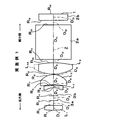•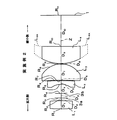•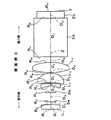•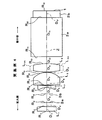•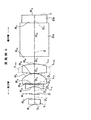•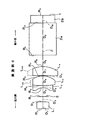•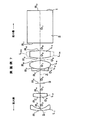•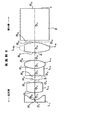••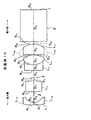•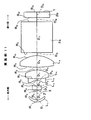•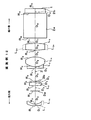•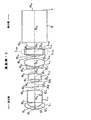•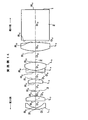•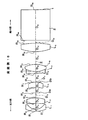•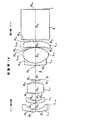•••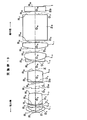•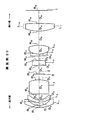•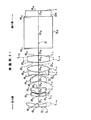•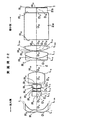••••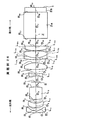•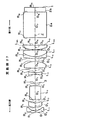••••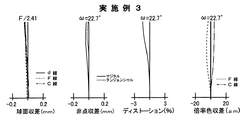••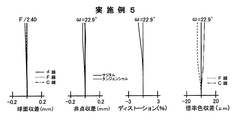•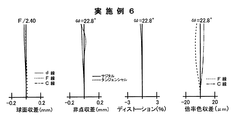•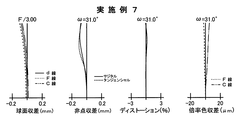•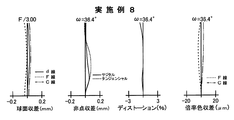•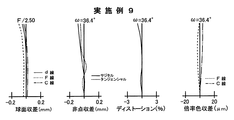••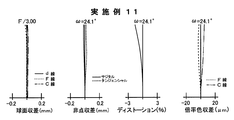•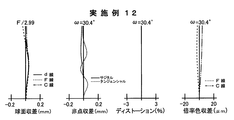••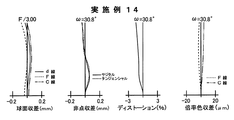•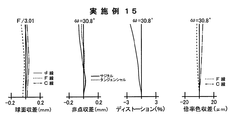•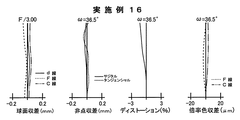•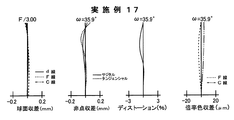•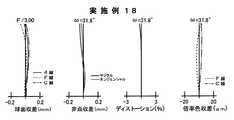•••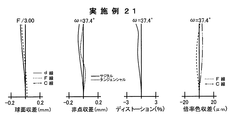•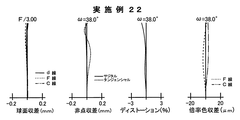••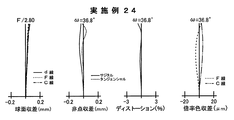•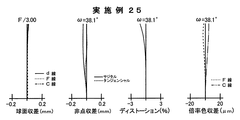•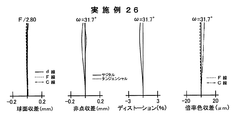•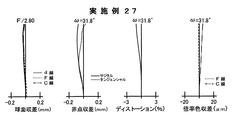•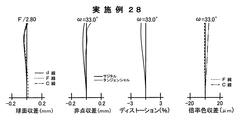••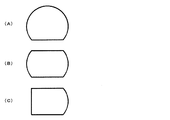## Classifications

• GPHYSICS
• G02OPTICS
• G02BOPTICAL ELEMENTS, SYSTEMS, OR APPARATUS
• G02B13/00Optical objectives specially designed for the purposes specified below
• G02B13/16Optical objectives specially designed for the purposes specified below for use in conjunction with image converters or intensifiers, or for use with projectors, e.g. objectives for projection TV
• GPHYSICS
• G02OPTICS
• G02BOPTICAL ELEMENTS, SYSTEMS, OR APPARATUS
• G02B9/00Optical objectives characterised both by the number of the components and their arrangements according to their sign, i.e. + or -
• G02B9/34Optical objectives characterised both by the number of the components and their arrangements according to their sign, i.e. + or - having four components only

## Abstract

A compact projection lens that can be adapted to a projection display device with excellent portability, has a wide angle so that the image size becomes large even in short-distance projection, and has good projection performance, and projection using the same A mold display device is obtained.
From A magnifying side in order, a first lens L 1 having a biconvex lens, and the aperture 3a and the aperture (or opening) 3b, and the second lens L 2 formed of a negative meniscus lens having a concave surface facing the magnification side , a third lens L 3, which is a positive meniscus lens having a convex surface on the reduction side, formed by arranging the fourth lens L 4 of aspherical which is a biconvex lens on the optical axis Z.
In addition, the minimum part of the length in the lens radial direction perpendicular to the optical axis of all lens elements is set to 15 mm or less, which satisfies the following conditional expression.
2.5 <β / S <10.0 (where S: maximum length of the enlarged image (inch), β: magnification)
[Selection] Figure 1

## Description

The present invention relates to a small projection lens for enlarging and projecting display information from a light valve such as a transmissive or reflective liquid crystal display element or DMD (digital micromirror device), and in particular, a so-called portable portability. The present invention relates to a small projection lens suitable for a projection display device of a type and a projection display device using the same.

Projection-type display devices using light valves such as liquid crystal display devices and DMD display devices are widely used. However, along with the popularization of personal computers, the light valves are rapidly becoming smaller and more precise. Since the demand for giving presentations using such a projection display device is also increasing, in recent years, a particularly small projection display device having excellent portability has been demanded. In the future, it may be possible to handle a projection display device as if it were a mobile phone or a flashlight. To that end, it is essential to further promote portability.

As a measure that can meet the above requirements, it is particularly effective to reduce the thickness so as to be smaller in the direction perpendicular to the optical axis of the projection optical system, generally in the thickness direction of the apparatus housing. It is important to reduce the outer diameter of all the lenses.

For the purpose of enhancing the portability of the apparatus, as a conventional technique for reducing the size of the projection lens, for example, the reduction side is made telecentric, as in the projection lenses described in Patent Documents 1 and 2, and color synthesis is performed. In addition, there is known a configuration in which a certain amount of space is secured in the lens back in order to separate illumination light and projection light.

Japanese Patent No. 3508011 JP-A-2005-84456

However, those described in Patent Documents 1 and 2 are difficult to project so that the angle of view is as small as about 50 degrees and the image size is large at a short distance.

Further, as described above, in Patent Documents 1 and 2, the reduction side is telecentric, and the lens back is secured to some extent. No measures for reducing the size of the lens are taken, and no positive measures for reducing the effective luminous flux are taken, including the reduction side lens and the enlargement side lens.

Furthermore, in order to achieve the above portability, it is effective to reduce the size of the light valve, but no proposal is made from such a viewpoint.

The present invention has been made in view of such circumstances, and can be adapted to a projection display device having excellent portability, and has a wide angle so as to increase the image size even in short-distance projection. It is an object of the present invention to provide a small projection lens having projection performance and a projection display device using the same.

The small projection lens according to the present invention is
In a small projection lens that projects the image information displayed at the reduction side conjugate position to the enlargement side conjugate position,
The minimum part of the length in the lens radial direction perpendicular to the optical axis of all the lens elements constituting the small projection lens is set to 15 mm or less,
The following conditional expression (1) is satisfied.
2.5 <β / S <10.0 (1)
here,
S: Maximum length of the enlarged image (inch)
β: magnification

The small projection lens preferably has any of the following six configurations.

That is, the first configuration is
The following conditional expressions (2) and (3) are satisfied.
2ω> 60 degrees (2)
FH <BH (3)
here,
2ω: Magnification-side angle of view FH: Maximum effective luminous flux height on the most magnified lens surface BH: Maximum effective luminous flux height on the most demagnifying lens surface

The second configuration is
The lens arranged on the most reduction side has a non-circular shape including an effective light beam passage region,
Furthermore, the following conditional expression (4) is satisfied.
Bf / f> 0.8 (4)
here,
Bf: Back focus on the reduction side f: Total system focal length

The third configuration is:
The lens arranged on the most reduction side has a non-circular shape including an effective light beam passage region,
Furthermore, the following conditional expressions (2 ′) and (4) are satisfied.
2ω> 35 degrees (2 ')
Bf / f> 0.8 (4)
here,
2ω: enlargement angle of view Bf: reduction-side back focus f: total focal length

The fourth configuration is:
The following conditional expressions (4) and (5) are satisfied.
Bf / f> 0.8 (4)
IH> TH (5)
here,
Bf: Back focus on the reduction side f: Focal length of the entire system IH: Maximum effective light beam height at the reduction side conjugate position TH: Maximum effective light beam height at the lens excluding the lens on the most reduction side

The fifth configuration is:
The following conditional expression (6) is satisfied.
20 <S / OBJ <65 (6)
here,
S: Maximum length of the enlarged image (inch)
OBJ: Expansion side projection distance (m)
β: magnification

The sixth configuration is:
The following conditional expressions (6 ′) and (7) are satisfied.
35 <S / OBJ <140 (6 ')
3.0 <S <10.0 (7)
here,
S: Maximum length of the enlarged image (inch)
OBJ: Expansion side projection distance (m)

Moreover, it is preferable that each of the small projection lenses is telecentric on the reduction side.

Next, a preferable aspect that can be applied to each of the small projection lenses will be described.

First, the first aspect is
In order from the magnifying side, a first lens having a positive refractive power, a second lens having a negative refracting power having a concave surface on the magnifying side, a third lens having a positive refracting power having a convex surface on the reducing side, and a positive lens It is constituted by a fourth lens having refractive power.

Next, the second aspect is:
In order from the enlargement side, the first lens having negative refractive power whose concave side is a concave surface, the second lens having positive refractive power whose convex side is a reduction side, the third lens having negative refractive power, and the convex side is a reduction side The fourth lens having a positive refractive power and the fifth lens having a positive refractive power.

Next, the third aspect is:
In order from the magnifying side, the first lens having a convex meniscus shape on the magnifying side, the second lens having a positive refractive power, the third lens having a negative refracting power on the magnifying side is concave, and the reducing side is a convex surface And a fourth lens having a positive refractive power and a fifth lens having a positive refractive power.

Next, the fourth aspect is
In order from the enlargement side, the first lens having negative refractive power having a concave surface on the reduction side, the second lens having positive refractive power having the convex side on the reduction side, and the first lens having positive refractive power having the convex side on the reduction side. 3 lenses, a fourth lens having a negative refractive power, a fifth lens having a positive refractive power whose convex side is a reduction side, and a sixth lens having a positive refractive power whose convex side is a magnification side. is there.

Next, the fifth aspect is
In order from the enlargement side, the first lens having negative refractive power having a concave surface on the reduction side, the second lens having negative refractive power having a concave surface on the reduction side, and the first lens having positive refractive power having a convex surface on the reduction side. 3 lenses, a fourth lens having a negative refractive power, a fifth lens having a positive refractive power whose convex side is a reduction side, and a sixth lens having a positive refractive power.

Next, the sixth aspect is
In order from the magnification side, the first lens having negative refractive power with the reduction side made concave, the second lens having negative refractive power made concave with the reduction side, the third lens having positive refractive power, and the convex side on the reduction side A fourth lens having a positive refractive power, a fifth lens having a negative refractive power, a sixth lens having a positive refractive power having a convex surface on the reduction side, and a positive refractive power having a convex surface on the magnification side It is comprised by the 7th lens which has.

Next, the seventh aspect is
In order from the magnification side, the first lens having negative refractive power, the second lens having positive refractive power, the third lens having negative refractive power, the fourth lens having positive refractive power, and the positive refractive power. A fifth lens having a negative refractive power, a seventh lens having a positive refractive power, and an eighth lens having a positive refractive power.

Next, the eighth aspect is
In order from the magnification side, the first lens having negative refractive power, the second lens having negative refractive power, the third lens having positive refractive power, the fourth lens having negative refractive power, and the positive refractive power. A fifth lens having a negative refractive power, a seventh lens having a positive refractive power, and an eighth lens having a positive refractive power.

In addition, a first projection display device of the present invention includes a light source, a plurality of light valves, an illumination optical unit that guides a light beam from the light source to the plurality of light valves, and any one of the small projection lenses. And light beams from the light source are modulated by the plurality of light valves, and the light-modulated lights are combined and projected onto the screen by the small projection lens.

A second projection display device of the present invention includes a light source, one light valve, an illumination optical unit that guides a light beam from the light source to the light valve, and any one of the small projection lenses described above. The light beam from the light source is optically modulated by the light valve and then projected onto a screen by the small projection lens.

The “aperture” described above only needs to have a function of restricting the passage of light flux, and includes a variable stop.

The “non-circular shape” means that the shape of each lens viewed from the light beam traveling direction is not circular.

According to the small projection lens of the present invention, in the small projection lens, the minimum portion of the length in the lens radial direction perpendicular to the optical axis of all lens elements constituting the small projection lens is 15 mm or less. Therefore, the lens system can be reduced in size. Furthermore, by satisfying conditional expression (1), it is possible to improve the illumination efficiency and increase the definition of the screen while suppressing an increase in the size of the apparatus.

Further, preferred embodiments of the small projection lens according to the present invention have the following effects.

That is, according to the first small projection lens, when the conditional expression (2) is satisfied, the angle of view on the enlargement side is defined to be larger than 60 degrees, and the image size becomes large even in short-distance projection. Configured as follows. Further, by satisfying conditional expression (3), the maximum effective light beam height on the most enlargement side lens surface is configured to be smaller than the maximum effective light beam height on the most reduction side lens surface. It is possible to prevent the diameter of the magnifying side lens from becoming too large with the increase in the size.

According to the second small projection lens, the reduction-side back focus is ensured by satisfying conditional expression (4), whereby separation of illumination light and projection light, and synthesis of color light are achieved. Etc. becomes easy. On the other hand, if the telecentricity on the reduction side is to be secured as the back focus becomes longer, the lens diameter on the reduction side becomes too large. Therefore, the lens arranged on the most reduction side is in a non-circular shape and a predetermined region other than the region necessary for light beam passage is cut off, so that the lens diameter on the reduction side can be prevented from becoming too large. .

Further, according to the third small projection lens, first, by satisfying conditional expression (2 ′), the angle of view on the wide-angle side is ensured to some extent, so that even with short-distance projection, the image size is to some extent. Configured to be large. Further, by satisfying conditional expression (4), the back focus on the reduction side is ensured, which facilitates separation of illumination light and projection light, synthesis of color light, and the like. However, if the conditional expression (2 ′) is satisfied, the diameter of the magnifying side lens becomes too large. If the conditional expression (4) is satisfied and the telecentricity on the reduction side is to be secured, The lens diameter becomes too large.

Therefore, the lens arranged on the most enlargement side and the lens arranged on the most reduction side are formed in a non-circular shape including an effective light flux passing region, so that the lens arranged on the most enlargement side and the lens arranged on the most reduction side are arranged. In addition, according to the fourth small projection lens, the back focus on the reduction side is secured by satisfying conditional expression (4). This facilitates separation of illumination light and projection light, synthesis of color light, and the like. However, if the conditional expression (4) is satisfied and the telecentricity on the reduction side is to be ensured, the lens diameter on the reduction side becomes too large. Therefore, if the conditional expression (5) is satisfied, in the lens excluding the lens on the most reduction side, the lens shape need not be a non-circular shape including the effective light beam passage region, and the sectional shape may be circular. Can be advantageous.

Further, according to the fifth small projection lens, when the conditional expression (6) is satisfied, the projection screen size (maximum length) and the projection distance can be set to appropriate values.

In addition, according to the sixth small projection lens, the brightness of the projection screen at the time of short-distance projection can be prevented from becoming too dark compared to the fifth small projection lens. That is, by satisfying conditional expression (7), the size of the light valve is not too small, and the projection screen can be made to be too dark.

Further, the projection display device of the present invention can be made a projection display device with excellent portability by using the small projection lens of the present invention, and the image size is sufficient even in short-distance projection. It is possible to have a wide angle so as to have a large size and a good projection performance.

It is a figure showing the structure of the small projection lens which concerns on Example 1 of this invention. It is a figure showing the structure of the small projection lens which concerns on Example 2 of this invention. It is a figure showing the structure of the small projection lens which concerns on Example 3 of this invention. It is a figure showing the structure of the small projection lens which concerns on Example 4 of this invention. It is a figure showing the structure of the small projection lens which concerns on Example 5 of this invention. It is a figure showing the structure of the small projection lens which concerns on Example 6 of this invention. It is a figure showing the structure of the small projection lens which concerns on Example 7 of this invention. It is a figure showing the structure of the small projection lens which concerns on Example 8 of this invention. It is a figure showing the structure of the small projection lens which concerns on Example 9 of this invention. It is a figure showing the structure of the small projection lens which concerns on Example 10 of this invention. It is a figure showing the structure of the small projection lens which concerns on Example 11 of this invention. It is a figure showing the structure of the small projection lens which concerns on Example 12 of this invention. It is a figure showing the structure of the small projection lens which concerns on Example 13 of this invention. It is a figure showing the structure of the small projection lens which concerns on Example 14 of this invention. It is a figure showing the structure of the small projection lens which concerns on Example 15 of this invention. It is a figure showing the structure of the small projection lens which concerns on Example 16 of this invention. It is a figure showing the structure of the small projection lens which concerns on Example 17 of this invention. It is a figure showing the structure of the small projection lens which concerns on Example 18 of this invention. It is a figure showing the structure of the small projection lens which concerns on Example 19 of this invention. It is a figure showing the structure of the small projection lens which concerns on Example 20 of this invention. It is a figure showing the structure of the small projection lens which concerns on Example 21 of this invention. It is a figure showing the structure of the small projection lens which concerns on Example 22 of this invention. It is a figure showing the structure of the small projection lens which concerns on Example 23 of this invention. It is a figure showing the structure of the small projection lens which concerns on Example 24 of this invention. It is a figure showing the structure of the small projection lens which concerns on Example 25 of this invention. It is a figure showing the structure of the small projection lens which concerns on Example 26 of this invention. It is a figure showing the structure of the small projection lens which concerns on Example 27 of this invention. It is a figure showing the structure of the small projection lens which concerns on Example 28 of this invention. FIG. 4 is a diagram illustrating various aberrations of the small projection lens according to Example 1; FIG. 6 is a diagram illustrating various aberrations of the small projection lens according to Example 2. FIG. 9 is a diagram illustrating all aberrations of the small projection lens according to Example 3; FIG. 10 is a diagram illustrating all aberrations of the small projection lens according to Example 4; FIG. 9 is a diagram illustrating all aberrations of the small projection lens according to Example 5; FIG. 9 is a diagram illustrating all aberrations of the small projection lens according to Example 6; FIG. 9 is a diagram illustrating various aberrations of the small projection lens according to Example 7. FIG. 10 is a diagram illustrating all aberrations of the small projection lens according to Example 8; FIG. 10 is a diagram illustrating all aberrations of the small projection lens according to Example 9; FIG. 10 is a diagram illustrating all aberrations of the small projection lens according to Example 10; FIG. 10 is a diagram illustrating all aberrations of the small projection lens according to Example 11; FIG. 14 is a diagram illustrating all aberrations of the small projection lens according to Example 12; FIG. 14 is a diagram illustrating all aberrations of the small projection lens according to Example 13; FIG. 19 is a diagram illustrating all aberrations of the small projection lens according to Example 14; FIG. 16 is a diagram illustrating all aberrations of the small projection lens according to Example 15; FIG. 20 is a diagram illustrating all aberrations of the small projection lens according to Example 16; FIG. 18 shows various aberrations of the small projection lens according to Example 17; FIG. 20 is a diagram illustrating all aberrations of the small projection lens according to Example 18; FIG. 20 is a diagram illustrating all aberrations of the small projection lens according to Example 19; FIG. 20 is a diagram illustrating all aberrations of the small projection lens according to Example 20; FIG. 20 is a diagram illustrating all aberrations of the small projection lens according to Example 21. FIG. 22 is a diagram illustrating all aberrations of the small projection lens according to Example 22; FIG. 24 is a diagram illustrating all aberrations of the small projection lens according to Example 23. FIG. 25 is a diagram illustrating all aberrations of the small projection lens according to Example 24. FIG. 27 is a diagram illustrating all aberrations of the small projection lens according to Example 25. FIG. 28 is a diagram illustrating all aberrations of the small projection lens according to Example 26. FIG. 42 shows various aberrations of the small projection lens according to Example 27. FIG. 42 shows various aberrations of the compact projection lens according to Example 28. It is a figure showing the schematic structure of the principal part of the projection type display apparatus of this invention. It is the schematic which shows the specific example of the non-circular shape of a lens when it sees from a light beam advancing direction.

Hereinafter, embodiments of the present invention will be described with reference to the drawings. FIG. 1 shows a small projection lens according to the present invention, and is a lens configuration diagram of Example 1 to be described later. In the figure, Z represents the optical axis.

The small projection lens according to the present embodiment is a small projection lens that projects image information displayed at the reduction-side conjugate position to the enlargement-side conjugate position, with respect to the optical axis of all lens elements constituting the small projection lens. The minimum portion of the length in the lens radial direction that is vertical is set to 15 mm or less, which satisfies the following conditional expression (1).
2.5 <β / S <10.0 (1)
here,
S: Maximum length of the enlarged image (inch)
β: magnification

The “maximum length” means the longest distance in the enlarged image, and is generally a diagonal length.

That is, the conditional expression (1) means that when various aberrations are not taken into account, it is necessary to use a panel size of 0.1 inch to 0.4 inch.

In addition, when it is out of the range of the conditional expression (1), it is difficult to improve the illumination efficiency and increase the screen definition while suppressing an increase in the size of the apparatus.

Further, the value of the “minimum portion of the length in the lens radial direction” is preferably “12 mm or less”, more preferably “10 mm or less”, instead of the “15 mm or less”.

In addition, the small projection lens according to the present embodiment preferably includes any one of the following six configurations.

That is, a preferable first configuration satisfies the following conditional expressions (2) and (3) in a small projection lens that projects image information displayed at the reduction-side conjugate position to the enlargement-side conjugate position.
2ω> 60 degrees (2)
FH <BH (3)
here,
2ω: Magnification-side angle of view FH: Maximum effective luminous flux height on the most magnified lens surface BH: Maximum effective luminous flux height on the most demagnifying lens surface

It should be noted that if it is below the lower limit of the conditional expression (2), it is difficult to make the image size sufficiently large when performing short distance projection.

Further, if the conditional expression (3) is not satisfied, it is difficult to suppress the diameter of the magnifying side lens from becoming too large as the angle of view increases.

In a preferred second configuration, in the small projection lens that projects the image information displayed at the reduction-side conjugate position to the enlargement-side conjugate position, the lens arranged closest to the reduction side includes a non-circular shape including an effective light beam passage region. Further, the following conditional expression (4) is satisfied.
Bf / f> 0.8 (4)
here,
Bf: Back focus on the reduction side f: Total system focal length

Note that if the lower limit of the conditional expression (4) is not reached, the reduction-side back focus is not secured, and it becomes difficult to separate illumination light and projection light, and to combine color light.

In addition, if the telecentricity on the reduction side is to be secured, the lens diameter on the reduction side becomes too large as the back focus becomes longer. The non-circular shape is included, and unnecessary lens portions are not provided (so-called D-cut is applied) to prevent the lens diameter on the reduction side from becoming too large.

In a preferred third configuration, in the small projection lens that projects the image information displayed at the reduction-side conjugate position to the enlargement-side conjugate position, the lens arranged closest to the reduction side includes a non-circular shape including an effective light beam passage region. Further, the following conditional expressions (2 ′) and (4) are satisfied.
2ω> 35 degrees (2 ')
Bf / f> 0.8 (4)
here,
2ω: enlargement angle of view Bf: reduction-side back focus f: total focal length

Note that if the value is equal to or lower than the lower limit of the conditional expression (2 ′), the angle of view on the wide angle side is not ensured, so that the image size cannot be sufficiently enlarged in the case of short-distance projection. On the other hand, if the lower limit of conditional expression (4) is not reached, the reduction-side back focus is not secured, and separation of illumination light and projection light, synthesis of color light, and the like become difficult.

A preferable fourth configuration satisfies the following conditional expressions (4) and (5) in a small projection lens that projects image information displayed at the reduction-side conjugate position to the enlargement-side conjugate position.
Bf / f> 0.8 (4)
IH> TH (5)
here,
Bf: Back focus on the reduction side f: Focal length of the entire system IH: Maximum effective light beam height at the reduction side conjugate position TH: Maximum effective light beam height at the lens excluding the lens on the most reduction side

Note that if the value is equal to or less than the lower limit of the conditional expression (4), the angle of view on the wide angle side is not ensured, so that the image size cannot be sufficiently enlarged in the case of short-distance projection. Further, if the conditional expression (5) is not satisfied, the reduction-side back focus is not ensured, and it becomes difficult to separate illumination light and projection light, and to combine color light.

A preferred fifth configuration satisfies the following conditional expression (6) in a small projection lens that projects image information displayed at the reduction-side conjugate position to the enlargement-side conjugate position.
20 <S / OBJ <65 (6)
here,
S: Maximum length of the enlarged image (inch)
OBJ: Expansion side projection distance (m)

The “maximum length” means the longest distance in the enlarged image, and is generally a diagonal length.

Note that if it falls outside the range of the conditional expression (6), it is difficult to set the projection screen size and the projection distance to appropriate values.

A preferable sixth configuration satisfies the following conditional expressions (6 ′) and (7) in a compact projection lens that projects image information displayed at the reduction-side conjugate position to the enlargement-side conjugate position. .
35 <S / OBJ <140 (6 ')
3.0 <S <10.0 (7)
here,
S: Maximum length of the enlarged image (inch)
OBJ: Expansion side projection distance (m)

The sixth small projection lens is suitable when mounted on, for example, an ultra-compact projection type image device that can be held by hand.

From the viewpoint of defining the conditional expression (6) as described above, it is preferable to satisfy the following conditional expression (6 ″) instead of the conditional expression (6).
30 <S / OBJ <65 (6 ″)

According to the sixth small projection lens, it is possible to obtain the same function and effect as the conditional expression (6) in the fifth small projection lens, and it is possible to have the function and effect according to the conditional expression (7). If this conditional expression (7) is not satisfied, it will be difficult to obtain an appropriate light valve size. That is, it is difficult to make the size of the light valve not too small and the projection image not too dark.

Each of the small projection lenses described above is composed of four to eight lenses (four lenses L 1 to L 4 in FIG. 1).

Furthermore, an aperture (which can be a stop) is disposed in the lens system.

In the small projection lens shown in FIG. 1, the light beam incident from the right side of the paper and provided with image information on the image display surface 1 of the light valve is reflected by the glass block 2a, various filters such as a low-pass filter and an infrared cut filter, The light enters the small projection lens through the cover glass 2b, and is enlarged and projected in the left direction on the paper surface by the small projection lens. Although only one image display surface 1 is shown in FIG. 1, in a projection display device, a light beam from a light source is separated into three primary color lights by a color separation optical system, and three light beams for each primary color light are used. It is possible to arrange a light valve so that a full color image can be displayed. Further, it is desirable that the reduction side is telecentric.

Specifically, a color synthesizing means (glass block) such as a cross dichroic prism, a DMD prism for separating illumination light and projection light, an LCOS PBS, and the like are disposed at the position of the glass block 2a. Is possible.

In addition, it is desirable that the small projection lens of the present embodiment has at least one aspherical surface, so that the resolution can be improved while reducing the number of lenses.

In the small projection lens of the present embodiment (particularly, a lens system having five or more lenses), the second lens and the third lens from the reduction side are joined to each other, so that the chromatic aberration, particularly the lateral chromatic aberration is good. It can be.

Further, in the small projection lens of the present embodiment, as shown in the examples described later, chromatic aberration can be improved by setting a conditional expression related to the Abbe number of a predetermined lens.

Further, specific lens configuration aspects according to embodiments of the present invention will be described below.

First, for example, as shown in FIGS. 1 to 6, the first mode is a first lens L 1 having a positive refractive power in order from the magnification side, and a second lens having a negative refractive power having a concave surface on the magnification side. L 2 is constituted by a third lens L 3 having a positive refractive power whose convex side is a reduction surface, and a fourth lens L 4 having a positive refractive power.

In this case, between the first lens L 1 and the second lens L 2, it is possible to arrange the opening to limit the passage of a light beam.

Further, it is possible that the fourth lens L 4 is configured to have an aspheric surface.

It is also possible to configure so as to satisfy the following conditional expressions (8) and (9).
ν d12 <45 (8)
ν d34 > 40 (9)
here,
ν d12 : Abbe number of each of the first lens L 1 and the second lens L 2 ν d34 : Abbe number of each of the third lens L 3 and the fourth lens L 4

Next, for example, as shown in FIGS. 7 to 10, the second mode is a positive first lens L 1 having negative refractive power having a concave surface on the reduction side and a convex surface on the reduction side, in order from the enlargement side. A second lens L 2 having a negative refractive power, a third lens L 3 having a negative refractive power, a fourth lens L 4 having a positive refractive power having a convex surface on the reduction side, and a fifth lens having a positive refractive power those constructed by the lens L 5.

In this case, between the second lens L 2 and the third lens L 3, it is possible to arrange the opening to limit the passage of a light beam.

It is also possible that the first lens L 1 is configured to have an aspheric surface.

Further, it is possible that the fifth lens L 5 is configured to have an aspheric surface.

It is also possible to said third lens L 3 and the fourth lens L 4 is configured to be joined together.

Further, it is possible to configure so as to satisfy the following conditional expression (10).
ν d4 −ν d3 > 35 (10)
here,
ν d4 : Abbe number of the fourth lens L 4 ν d3 : Abbe number of the third lens L 3

Next, as shown in FIG. 11 and FIG. 12, for example, the third mode is a first lens L 1 having a positive refractive power in which, from the enlargement side, in order from the enlargement side, a convex surface and a meniscus shape. A second lens L 2 having a power, a third lens L 3 having a negative refracting power having a concave surface on the enlargement side, a fourth lens L 4 having a positive refracting power having a convex surface on the reduction side, and a positive refracting power. with those constituted by the fifth lens L 5.

In this case, between the first lens L 1 and the second lens L 2, it is possible to arrange the opening to limit the passage of a light beam.

It is also possible that the first lens L 1 is configured to have an aspheric surface.

Further, it is possible that the fifth lens L 5 is configured to have an aspheric surface.

It is also possible to said third lens L 3 and the fourth lens L 4 is configured to be joined together.

Further, it is possible to configure so as to satisfy the following conditional expression (10).
ν d4 −ν d3 > 35 (10)
here,
ν d4 : Abbe number of the fourth lens L 4 ν d3 : Abbe number of the third lens L 3

Next, as shown in, for example, FIGS. 13 to 15, the fourth mode is a positive first lens L 1 having negative refractive power having a concave surface on the reduction side and a convex surface on the reduction side, in order from the enlargement side. A second lens L 2 having a refractive power, a third lens L 3 having a positive refractive power whose convex side is a reduction side, a fourth lens L 4 having a negative refractive power, and a positive refraction having a convex side on a reduction side The fifth lens L 5 having power and the sixth lens L 6 having positive refractive power whose convex side is a convex surface are used.

In this case, between the second lens L 2 and third lens L 3, it is possible to arrange the opening to limit the passage of a light beam.

It is also possible that the first lens L 1 is configured to have an aspheric surface.

It is also possible to said fourth lens L 4 and the fifth lens L 5 is configured to be joined together.

Next, in the fifth mode, for example, as shown in FIGS. 16 to 18, in order from the enlargement side, the first lens L 1 having negative refractive power having a concave surface on the reduction side and a negative surface having a concave surface on the reduction side. A second lens L 2 having a refractive power, a third lens L 3 having a positive refractive power whose convex side is a reduction side, a fourth lens L 4 having a negative refractive power, and a positive refraction having a convex side on a reduction side The fifth lens L 5 having power and the sixth lens L 6 having positive refractive power are configured.

Further, between the third lens L 3 and the fourth lens L 4, it is possible to arrange the opening to limit the passage of a light beam.

It is also possible that the second lens L 2 is configured to have an aspheric surface.

It is also possible that the sixth lens L 6 is configured to have an aspheric surface.

It is also possible to said fourth lens L 4 and the fifth lens L 5 is configured to be joined together.

Moreover, in the said 4th aspect and a 5th aspect, it is possible to comprise so that the following conditional expressions (11) may be satisfied.
ν d5 −ν d4 > 35 (11)
here,
ν d5 : Abbe number of the fifth lens L 5 ν d4 : Abbe number of the fourth lens L 4

Next, for example, as shown in FIGS. 19 to 21, the sixth mode is the first lens L 1 having negative refractive power in which the reduction side is concave in order from the enlargement side, and the negative side in which the reduction side is concave. A second lens L 2 having a positive refractive power, a third lens L 3 having a positive refractive power, a fourth lens L 4 having a positive refractive power having a convex surface on the reduction side, and a fifth lens L having a negative refractive power. 5. A sixth lens L 6 having a positive refractive power whose convex side is a reduction surface and a seventh lens L 7 having a positive refractive power whose convex side is a magnification side.

In this case, between the third lens L 3 and the fourth lens L 4, it is possible to arrange the opening to limit the passage of a light beam.

It is also possible that the second lens L 2 is configured to have an aspheric surface.

It is also possible to said fifth lens L 5 and the sixth lens L 6 is configured to be joined together.

Next, for example, as shown in FIGS. 22 to 24, the seventh aspect includes, in order from the enlargement side, a first lens L 1 having a negative refractive power, a second lens L 2 having a positive refractive power, a negative A third lens L 3 having a refractive power, a fourth lens L 4 having a positive refractive power, a fifth lens L 5 having a positive refractive power, a sixth lens L 6 having a negative refractive power, and a positive refraction. This is constituted by a seventh lens L 7 having a power and an eighth lens L 8 having a positive refractive power.

In this case, the first lens L 1 may be a plastic aspherical lens.

Further, between the second lens L 2 and the fifth lens L 5, it is possible to arrange the opening to limit the passage of a light beam.

It is also possible to said sixth lens L 6 and the seventh lens L 7 is configured to be joined together.

Next, for example, as illustrated in FIGS. 25 to 28, the eighth aspect includes a first lens L 1 having negative refractive power, a second lens L 2 having negative refractive power, A third lens L 3 having a negative refractive power, a fourth lens L 4 having a negative refractive power, a fifth lens L 5 having a positive refractive power, a sixth lens L 6 having a negative refractive power, and a positive refraction. This is constituted by a seventh lens L 7 having a power and an eighth lens L 8 having a positive refractive power.

In this case, the second lens L 2 is may be a plastic aspherical lens.

Between the third lens L 3 of the fourth lens L 4, it is possible to arrange the opening to limit the passage of a light beam.

It is also possible to said sixth lens L 6 and the seventh lens L 7 is configured to be joined together.

Next, an embodiment of a projection display device according to the present invention will be described. FIG. 57 is a block diagram showing an example of a main part (illumination optical system 10) of the projection display apparatus according to one embodiment of the present invention.

As shown in FIG. 57, the illumination optical system 10 includes transmissive liquid crystal panels 11a to 11c as light valves, dichroic mirrors 12 and 13 for color separation, a cross dichroic prism 14 for color composition, and a condenser. Lenses 16a to 16c and total reflection mirrors 18a to 18c are provided. Although the illustration of the front stage of the dichroic mirror 12 is omitted, the white light from the light source passes through the illumination optical unit and the liquid crystal panels 11a to 11c respectively correspond to the three color light beams (G light, B light, and R light). Then, the light is modulated and projected onto a screen by a small projection lens.

Since this projection type display device uses the small projection lens according to the present invention, it is possible to obtain a high-resolution large screen with various aberrations well corrected with a compact size.

In order to promote further compactness, the light valve is a single plate, for example, RGB color images are sequentially displayed on the light valve, and in synchronization with this, corresponding color light is emitted from a light source composed of RGB color LEDs. It is preferable to output. Thereby, the dichroic mirrors 12 and 13 for color separation, the cross dichroic prism 14 for color synthesis, the total reflection mirrors 18a to 18c, and the like described above can be omitted.

Specific examples of the small projection lens according to the present invention will be described below. In addition, in each Example, the same code | symbol is attached | subjected about the member which makes mutually the same effect.
(First aspect)

As shown in FIG. 1, the projection lens according to Example 1 has a first lens L 1 composed of a biconvex lens, an aperture 3 a and an aperture (or aperture) 3 b, and a concave surface directed toward the magnification side in order from the magnification side. negative and the second lens L 2 formed of a meniscus lens, a third lens L 3, which is a positive meniscus lens having a convex surface on the reduction side, the fourth lens has been aspherical on the optical axis Z a biconvex lens L consisting of 4.

Further, the fourth lens L 4 arranged on the most reduction side is formed into a non-circular shape including an effective light beam passage region, and an unnecessary lens portion (portion located above the drawing) L 4A is not provided (so-called D To prevent the lens diameter on the reduction side from becoming too large.

Aspherical shape of the both surfaces of the fourth lens L 4 are defined by the aspheric expression below.

FIG. 1 also shows an image display surface 1 of the light valve, a glass block 2a, and a cover glass 2b of the light valve.

The radius of curvature R of each lens surface of the projection lens according to Example 1, the center thickness of each lens, the air space between the lenses (hereinafter referred to as “axial surface space”) D, and the refractive index N d of each lens at the d-line. The values of the Abbe number ν d are shown in the upper part of Table 1. In Table 1 and the following table, the surface number numbers indicate the order from the enlargement side, and the surface marked with * on the right side of the surface number is an aspheric surface. The lower part of Table 1 shows aspheric coefficients representing the respective aspheric surfaces.

Further, in Example 1 and Examples 2 to 28 below, the curvature radius R of these aspheric surfaces is shown as the value of the curvature radius R on the optical axis Z in each table. In order to make the drawing easier to see, the leader line is not necessarily drawn from the intersection with the optical axis Z.

As shown in Table 29, the projection lens of Example 1 satisfies the conditional expressions (1), (3) to (6), (8), (9), (2 ′), and (6 ″). ing.

In Example 1, the focal length f of the entire system is set to 10.6, the F number Fno. Is set to 3.01, and the total angle of view 2ω is set to 48.2 (degrees).

The configuration of the projection lens according to Example 2 is as shown in FIG. 2, and the lens configuration itself is substantially the same as that of Example 1. However, since the light valve is configured to correspond to those of a single plate, between the image display surface 1 of the fourth lens L 4 and the light valve, for color synthesis as in Example 1 The glass block 2a is not arranged. Further, the cover glass 2b of the light valve is also omitted.

The fourth lens L 4 arranged on the most reduction side has a non-circular shape including an effective light beam passage region, and an unnecessary lens portion (portion positioned in the vertical direction in the drawing) L 4A is not provided (so-called (D cut) to prevent the lens diameter on the reduction side from becoming too large.

Moreover, the aspherical shape of the both surfaces of the fourth lens L 4 are defined by the aspheric expression shown above.

Table 2 shows the values of the radius of curvature R of each lens surface, the axial top surface distance D, the refractive index N d of each lens at the d-line, and the Abbe number ν d of the projection lens according to Example 2. In addition, the lower part of Table 2 shows the aspheric coefficients representing the respective aspheric surfaces.

As shown in Table 29, the projection lens according to Example 2 is configured to satisfy the conditional expressions (1) to (9), (2 ′), (6 ′), and (6 ″). Yes.

In Example 2, the focal length f of the entire system is set to 5.9, the F number Fno. Is 2.51, and the total angle of view 2ω is 61.8 (degrees).

The configuration of the projection lens according to Example 3 is as shown in FIG. 3, and the lens configuration itself is substantially the same as that of Example 1. However, the second lens L 2 is a biconcave lens.

In addition, what is called D cut in the said Example 1 is not given.

Moreover, the aspherical shape of the both surfaces of the fourth lens L 4 are defined by the aspheric expression shown above.

Table 3 shows values of the curvature radius R of each lens surface, the axial top surface distance D, the refractive index N d of each lens at the d-line, and the Abbe number ν d of the projection lens according to Example 3. In addition, the lower part of Table 3 shows the aspheric coefficients representing the respective aspheric surfaces.

As shown in Table 29, the projection lens according to Example 3 satisfies the conditional expressions (1), (3) to (6), (8), (9), (2 ′), and (6 ″). It is configured to satisfy.

In Example 3, the focal length f of the entire system is set to 9.9, the F number Fno. Is set to 2.51, and the total angle of view 2ω is set to 45.4 (degrees).

The configuration of the projection lens according to Example 4 is as shown in FIG. 4, and the lens configuration itself is substantially the same as that of Example 1. However, the second lens L 2 is a biconcave lens, a third lens L 3 is a biconvex lens.

The fourth lens L 4 arranged on the most reduction side has a non-circular shape including an effective light beam passage region, and an unnecessary lens portion (portion positioned in the vertical direction in the drawing) L 4A is not provided (so-called (D cut) to prevent the lens diameter on the reduction side from becoming too large.

Moreover, the aspherical shape of the both surfaces of the fourth lens L 4 are defined by the aspheric expression shown above.

The values of the curvature radius R of each lens surface, the axial top surface distance D, the refractive index N d of each lens at the d-line and the Abbe number ν d of the projection lens according to Example 4 are shown in the upper part of Table 4. In addition, the lower part of Table 4 shows the aspheric coefficients representing the respective aspheric surfaces.

As shown in Table 29, the projection lens according to Example 4 has the above conditional expressions (1), (3), (4), (6), (8), (9), and (2 ′). , (6 ″) is satisfied.

In Example 4, the focal length f of the entire system is set to 9.9, the F number Fno. Is set to 2.51, and the total angle of view 2ω is set to 45.4 (degrees).

The configuration of the projection lens according to Example 5 is as shown in FIG. 5, and the lens configuration itself is substantially the same as that of Example 1. However, the second lens L 2 is a biconcave lens. The mask 3 is distribution only one between the first lens L 1 and second lens L 2.

The fourth lens L 4 arranged on the most reduction side has a non-circular shape including an effective light beam passage region, and an unnecessary lens portion (portion positioned in the vertical direction in the drawing) L 4A is not provided (so-called (D cut) to prevent the lens diameter on the reduction side from becoming too large.

Moreover, the aspherical shape of the both surfaces of the fourth lens L 4 are defined by the aspheric expression shown above.

Table 5 shows the values of the curvature radius R of each lens surface, the axial top surface distance D, the refractive index N d of each lens at the d-line, and the Abbe number ν d of the projection lens according to Example 5. In addition, the lower part of Table 5 shows the aspheric coefficients representing the respective aspheric surfaces.

As shown in Table 29, the projection lens according to Example 5 has the above conditional expressions (1), (3), (4), (6), (8), (9), (2 ′). , (6 ″) is satisfied.

In Example 5, the focal length f of the entire system is set to 7.8, the F number Fno. Is set to 2.40, and the total angle of view 2ω is set to 45.8 (degrees).

The configuration of the projection lens according to Example 6 is as shown in FIG. 6, and the lens configuration itself is substantially the same as that of Example 1. However, the first lens L 1 is a positive meniscus lens having a concave surface directed toward the magnification side, a second lens L 2 is a biconcave lens, a third lens L 3 is a biconvex lens, and a second lens L 2 The third lens L 3 is different in that it is cemented with each other.

The fourth lens L 4 arranged closest to the reduction side and the third lens L 3 adjacent to the enlargement side have a non-circular shape including an effective light beam passage region, and an unnecessary lens portion (positioned in the upward direction in the drawing). parts) L 3A, not be provided with the L 4A (subjected to so-called D-cut), thereby preventing the lens diameter on the reduction side becomes too large.

Moreover, the aspherical shape of the both surfaces of the fourth lens L 4 are defined by the aspheric expression shown above.

The values of the curvature radius R of each lens surface, the axial top surface distance D, the refractive index N d of each lens at the d-line and the Abbe number ν d of the projection lens according to Example 6 are shown in the upper part of Table 6. In addition, the lower part of Table 6 shows the aspheric coefficients representing the respective aspheric surfaces.

As shown in Table 6, the projection lens according to Example 6 has the conditional expressions (1), (3), (4), (6), (8), (9), (2 ′), (6 ″ ″).

In Example 6, the focal length f of the entire system is set to 7.8, the F number Fno. Is set to 2.40, and the total angle of view 2ω is set to 45.6 (degrees).
(Second aspect)

As shown in FIG. 7, the projection lens according to Example 7 includes, in order from the magnification side, a first lens L 1 composed of a negative meniscus double-sided aspheric lens with a convex surface facing the magnification side, and a biconvex lens. a second lens L 2, and (can also be a variable diaphragm) 3 opening, a cemented lens formed by cementing a fourth lens L 4 and a third lens L 3 and a biconvex lens is a biconcave lens, a biconvex lens consisting fifth lens L 5 formed of.

Further, the fifth lens L 5 arranged on the most reduction side has a non-circular shape including an effective light beam passage region, and an unnecessary lens portion (portion positioned in the vertical direction of the drawing) L 5A is not provided (so-called D cut is performed) to prevent the outer diameter of the lens on the reduction side from becoming too large.

Aspherical shape of the surfaces of the first lens L 1 is defined by the aspheric equation shown above.

Further, FIG. 7 shows an image display surface 1 and a glass block 2 of the light valve.

Table 7 shows the values of the curvature radius R of each lens surface, the axial top surface distance D, the refractive index N d of each lens at the d-line, and the Abbe number ν d of the projection lens according to Example 7.

As shown in Table 29, the conditional expressions (1) to (6), (10), (2 ′), (6 ′), and (6 ″) are satisfied in Example 7.

In Example 7, the focal length f of the entire system is set to 7.8, the F number Fno. Is set to 3.00, and the total angle of view 2ω is set to 62.0 (degrees).

Structure of a projection lens according to Example 8 is as shown in FIG. 8, the lens configuration itself is substantially the same as in Example 7, the first lens L 1 is a bi-concave aspherical lens Further, the fifth lens L 5 is different from the first lens L 1 in that it is composed of a double-sided aspheric lens.

The fifth lens L 5 arranged on the most reduction side has a non-circular shape including an effective light beam passage region, and an unnecessary lens portion (portion located in the upward direction in the drawing) L 5A is not provided (so-called (D cut) to prevent the lens diameter on the reduction side from becoming too large.

Moreover, the aspherical shape of the surfaces of the first lens L 1 and the fifth lens L 5 is defined by the aspheric equation shown above.

The values of the curvature radius R of each lens surface, the axial top surface distance D, the refractive index N d of each lens at the d-line and the Abbe number ν d of the projection lens according to Example 8 are shown in the upper part of Table 8. In addition, the lower part of Table 8 shows aspheric coefficients representing the respective aspheric surfaces.

As shown in Table 29, the projection lens according to Example 8 satisfies the conditional expressions (1) to (6), (10), (2 ′), (6 ′), and (6 ″). It is configured.

In Example 8, the focal length f of the entire system is set to 6.4, the F number Fno. Is set to 3.00, and the total angle of view 2ω is set to 72.8 (degrees).

Structure of a projection lens according to Example 9 is as shown in FIG. 9, the lens configuration itself is substantially the same as in Example 7, in addition to the first lens L 1, the fifth lens L 5 It is different in that it is a double-sided aspheric lens.

Aspherical shape of the surfaces of the first lens L 1 and the fifth lens L 5 is defined by the aspheric equation shown above.

Table 9 shows the values of the curvature radius R of each lens surface, the axial top surface distance D, the refractive index N d of each lens at the d-line, and the Abbe number ν d of the projection lens according to Example 9. In addition, the lower part of Table 9 shows aspheric coefficients representing the respective aspheric surfaces.

As shown in Table 29, the projection lens according to Example 9 satisfies the conditional expressions (1) to (6), (10), (2 ′), (6 ′), and (6 ″). It is configured.

In Example 9, the focal length f of the entire system is set to 6.4, the F number Fno. Is 2.50, and the total angle of view 2ω is 72.8 (degrees).

The structure of a projection lens according to Example 10, is as shown in FIG. 10, the lens configuration itself is substantially the same as in Example 7, in addition to the first lens L 1 fifth lens L 5 is also a bi- The first lens L 1 is composed of a biconcave double-sided aspheric lens on the optical axis Z, and the third lens L 3 is composed of a negative meniscus lens with the concave surface facing the reduction side. Furthermore, the fifth lens L 5 is different in that it is composed of a positive meniscus double-sided aspheric lens with the convex surface facing the reduction side on the optical axis Z.

Further, the first lens L 1 arranged on the most enlargement side and the fifth lens L 5 arranged on the most reduction side do not provide unnecessary lens portions (portions positioned in the vertical direction of the drawing) L 1A and L 5A . In this way (so-called D-cut is applied), the lens diameter on the reduction side is prevented from becoming too large.

Aspherical shape of the surfaces of the first lens L 1 and the fifth lens L 5 is defined by the above-mentioned aspheric surface expression.

The values of the curvature radius R of each lens surface, the axial top surface distance D, the refractive index N d of each lens at the d-line and the Abbe number ν d of the projection lens according to Example 10 are shown in the upper part of Table 10. In addition, the lower part of Table 10 shows aspheric coefficients representing the respective aspheric surfaces.

As shown in Table 29, the projection lens according to Example 10 is configured to satisfy the conditional expressions (1) to (5), (7), (10), (2 ′), and (6 ′). Has been.

In addition, since the projection lens of Example 10 satisfies the conditional expressions (6 ′) and (7) at the same time, it can be mounted on an ultra-small handheld projection display device. .

In Example 10, the focal length f of the entire system is set to 5.1, the F number Fno. Is set to 3.00, and the total angle of view 2ω is set to 86.4 (degrees).
(Third aspect)

Structure of a projection lens according to Example 11 is as shown in FIG. 11, the first lens L 1, which is a positive meniscus lens having a convex surface on the enlargement side, an aperture (or stop) 3, the magnification side a second lens L 2, which is a positive meniscus lens having a convex surface directed toward, a cemented lens formed by cementing a fourth lens L 4 and a third lens L 3 and a biconvex lens and a bi-concave lens, a double convex non composed by disposing the fifth lens L 5 formed of a spherical lens.

Aspherical shape of the surfaces of the fifth lens L 5 is defined by the aspheric equation shown above.

FIG. 11 also shows the image display surface 1 of the light valve, the glass block 2a, and the cover glass 2b of the light valve.

The values of the curvature radius R of each lens surface, the axial top surface distance D, the refractive index N d of each lens at the d-line and the Abbe number ν d of the projection lens according to Example 11 are shown in the upper part of Table 11. The lower part of Table 11 shows aspheric coefficients representing the respective aspheric surfaces.

As shown in Table 29, the compact projection lens according to Example 11 is configured to satisfy the conditional expressions (1), (3) to (6), (2 ′).

In Example 11, the focal length f of the entire system is set to 10.6, the F number Fno. Is set to 3.00, and the total angle of view 2ω is set to 48.2 (degrees). Therefore, the wide angle of view is as small as five lenses.

Structure of a projection lens according to Example 12 is as shown in FIG. 12, the lens configuration itself is substantially the same as Example 11, the first lens L 1 is negative for a convex surface on the enlargement side in that consists of a meniscus-shaped aspherical lens, and the second lens L 2 is, in that a biconvex lens, in addition to the mask 3a, also between the second lens L 2 and third lens L 3 The difference is that a mask (which can be a variable aperture) 3b is provided.

Further, the fifth lens L 5 arranged on the most reduction side has a non-circular shape including an effective light beam passage region, and an unnecessary lens portion (portion positioned in the vertical direction of the drawing) L 5A is not provided (so-called The first lens L 1 disposed on the most enlarged side is made into a non-circular shape including an effective light beam passage region, and an unnecessary lens portion (portion located in the downward direction in the drawing) L 1A is formed. The lens diameter on the reduction side is prevented from becoming too large by not providing (so-called D-cut).

Moreover, the aspherical shape of the surfaces of the first lens L 1 and the fifth lens L 5 is defined by the aspheric equation shown above.

The values of the curvature radius R of each lens surface, the axial top surface distance D, the refractive index N d of each lens at the d-line and the Abbe number ν d of the projection lens according to Example 12 are shown in the upper part of Table 12. In addition, the lower part of Table 12 shows aspheric coefficients representing the respective aspheric surfaces.

As shown in Table 29, the projection lens according to Example 12 satisfies the above conditional expressions (1) to (6), (10), (2 ′), (6 ′), and (6 ″). It is configured.

In Example 12, the focal length f of the entire system is set to 7.9, the F number Fno. Is 2.99, and the total angle of view 2ω is 60.8 (degrees). Therefore, the number of lenses is as small as 5, and a wide angle of view is provided for each stage.
(Fourth aspect)

Projection lens according to Example 13 is as shown in FIG. 13, in order from the magnification side, a first lens L 1 of the aspherical consisting of a negative meniscus lens having a convex surface facing the magnification side, a convex surface on the reduction side a positive second lens L 2 formed of meniscus lens, an aperture 3a and the aperture (or opening) 3b, and the third lens L 3, which is a positive meniscus lens having a convex surface facing the reduction side, a biconcave lens a fourth lens L 4 and the fifth lens L 5 formed by bonding a cemented lens consisting of a biconvex lens comprising, consisting by arranging a sixth lens L 6, which is a biconvex lens.

The sixth lens L 6 arranged on the most reduction side has a non-circular shape including an effective light beam passage region, and unnecessary lens portions (a portion positioned in the vertical direction of the drawing and both side portions positioned in the depth direction of the drawing) L 6A is not provided (so-called D-cut is applied to the four-direction portion) to prevent the lens diameter on the reduction side from becoming too large.

Aspherical shape of the surfaces of the first lens L 1 is defined by the aspheric equation shown above.

FIG. 13 shows an image display surface 1 and a glass block 2 of the light valve.

The values of the curvature radius R of each lens surface, the axial top surface distance D, the refractive index N d of each lens at the d-line and the Abbe number ν d of the projection lens according to Example 13 are shown in the upper part of Table 13. Also, the lower part of Table 13 shows the aspheric coefficients representing the respective aspheric surfaces.

In the present embodiment and the following embodiments, the curvature radius R of these aspheric surfaces is shown as the value of the curvature radius R on the optical axis Z in each table. In order to make it easier to see, some leader lines are not necessarily drawn from the intersection with the optical axis Z.

As shown in Table 29, the projection lens according to Example 13 satisfies the conditional expressions (1) to (6), (11), and (2 ′).

In Example 13, the focal length f of the entire system is set to 6.4, the F number Fno. Is set to 3.00, and the total angle of view 2ω is set to 73.2 (degrees).

The configuration of the projection lens according to Example 14 is as shown in FIG. 14, and the lens configuration itself is substantially the same as that of Example 13, except that only one aperture (or stop) 3 is mainly provided. It is different in point.

Aspherical shape of the surfaces of the first lens L 1 is defined by the aspheric equation shown above.

Table 14 shows the values of the curvature radius R of each lens surface, the axial top surface distance D, the refractive index N d of each lens at the d-line, and the Abbe number ν d of the projection lens according to Example 14. In addition, an aspheric coefficient representing each aspheric surface is shown in the lower part of Table 14.

As shown in Table 29, the projection lens according to Example 14 satisfied the conditional expressions (1) to (6), (11), (2 ′), (6 ′), (6 ″) Yes.

In Example 14, the focal length f of the entire system is set to 7.9, the F number Fno. Is set to 3.00, and the total angle of view 2ω is set to 61.6 (degrees).

Structure of a projection lens according to Example 15 is as shown in FIG. 15, the lens configuration is substantially the same as Example 13, primarily, one opening (or aperture) 3b is, the third lens L 3 It is different in that provided between the fourth lens L 4.

Aspherical shape of the surfaces of the first lens L 1 is defined by the aspheric equation shown above.

FIG. 15 shows the image display surface 1 and the glass block 2 of the light valve.

The values of the curvature radius R of each lens surface, the axial top surface distance D, the refractive index N d of each lens at the d-line and the Abbe number ν d of the projection lens according to Example 15 are shown in the upper part of Table 15. In addition, an aspheric coefficient representing each aspheric surface is shown in the lower part of Table 15.

As shown in Table 29, the projection lens according to Example 1 satisfies the conditional expressions (1) to (7), (11), (2 ′), (6 ′), and (6 ″). It is configured.

The projection lens of the fifteenth embodiment particularly satisfies the conditional expressions (6 ′) and (7) at the same time, so that it can be mounted on an ultra-small handheld projection display device. .

In Example 1, the focal length f of the entire system is set to 8.0, the F number Fno. Is set to 3.01, and the total angle of view 2ω is set to 61.6 (degrees).
(Fifth aspect)

Negative projection lens according to Example 16 is as shown in FIG. 16, directed in order from the magnification side, a first lens L 1 composed of a negative meniscus lens having a convex surface facing the magnification side, a concave surface on the reduction side a second lens L 2 formed of double-sided aspheric lens of the meniscus shape, a third lens L 3, which is a biconvex lens, an aperture 3 (or variable aperture), a negative meniscus lens having a concave surface facing the reduction side a fourth lens L 4 and the cemented lens formed by cementing a fifth lens L 5 which is a biconvex lens, made by arranging a sixth lens L 6 comprising a positive bi-aspherical lens.

The sixth lens L 6 arranged on the most reduction side, the first lens L 1 arranged on the most enlargement side, and the fifth lens L 5 have a non-circular shape including an effective light beam passage region, which is unnecessary. The lens portion (portion located in the upper or lower direction of the drawing) L 6A , L 1A , and L 5A is not provided (so-called D-cut is applied) to prevent the lens diameter on the reduction side from becoming too large. ing.

Both surfaces aspheric shape of the second lens L 2 and the sixth lens L 6 are defined by the aspheric equation shown above.

FIG. 16 shows the image display surface 1 and the glass block 2 of the light valve.

The values of the curvature radius R of each lens surface, the axial top surface distance D, the refractive index N d of each lens at the d-line and the Abbe number ν d of the projection lens according to Example 16 are shown in the upper part of Table 16. In addition, the lower part of Table 16 shows aspheric coefficients representing the respective aspheric surfaces.

As shown in Table 29, the projection lens according to Example 16 has conditional expressions (1) to (4), (6), (11), (2 ′), (6 ′), and (6 ″). Is satisfied.

In Example 16, the focal length f of the entire system is set to 6.4, the F number Fno. Is set to 3.00, and the total angle of view 2ω is set to 73.0 (degrees).

Structure of a projection lens according to Example 17 is as shown in FIG. 17 has substantially the same manner as in Example 16, are mainly, second lens L 2 is a biconcave aspherical lens Point, the fourth lens L 4 is a biconcave lens, and two apertures (or variable diaphragms) 3 a and 3 b are provided between the third lens L 3 and the fourth lens L 4. Are different.

Both surfaces aspheric shape of the second lens L 2 and the sixth lens L 6 are defined by the aspheric equation shown above.

The values of the curvature radius R of each lens surface, the axial top surface distance D, the refractive index N d of each lens at the d-line and the Abbe number ν d of the projection lens according to Example 17 are shown in the upper part of Table 17. In addition, the lower part of Table 17 shows the aspheric coefficients representing the respective aspheric surfaces.

As shown in Table 29, the projection lens according to Example 17 satisfied the conditional expressions (1) to (6), (11), (2 ′), (6 ′), (6 ″) Yes.

In Example 17, the focal length f of the entire system is set to 6.5, the F number Fno. Is set to 3.00, and the total angle of view 2ω is set to 71.8 (degrees).

The structure of a projection lens according to Example 18, is as shown in FIG. 18 is similar to Example 17 above, primarily, both surfaces of the negative meniscus second lens L 2 is that a concave surface facing the reduction side non It is different in that it is a spherical lens.

Both surfaces aspheric shape of the second lens L 2 and the sixth lens L 6 are defined by the aspheric equation shown above.

The values of the curvature radius R of each lens surface, the axial top surface distance D, the refractive index N d of each lens at the d-line and the Abbe number ν d of the projection lens according to Example 18 are shown in the upper part of Table 18. In addition, an aspheric coefficient representing each aspheric surface is shown in the lower part of Table 18.

As shown in Table 29, the projection lens according to Example 18 satisfied the conditional expressions (1) to (7), (11), (2 ′), (6 ′), (6 ″) Yes.

The projection lens of Example 18 particularly satisfies the conditional expressions (6 ′) and (7) at the same time. Therefore, the projection lens can be mounted on an ultra-small handheld projection display device. .

In Example 18, the focal length f of the entire system is set to 7.6, the F number Fno. Is set to 3.00, and the total angle of view 2ω is set to 63.6 (degrees).
(Sixth aspect)

Negative projection lens according to Example 19 is as shown in FIG. 19, directed in order from the magnification side, a first lens L 1 composed of a negative meniscus lens having a convex surface facing the magnification side, a concave surface on the reduction side A positive meniscus lens having a convex surface facing the reduction side, a second lens L 2 made of a meniscus double-sided aspheric lens, a third lens L 3 made of a biconvex lens, apertures (or variable diaphragms) 3 a, 3 b. a fourth lens L 4 made of a cemented lens formed by cementing a sixth lens L 6 made of the fifth lens L 5 and a biconvex lens comprising a plano-concave lens having a concave surface facing the reduction side, seventh biconvex lens the lens L 7 formed by arranged.

Aspherical shape of the surfaces of the second lens L 2 is defined by the aspheric equation shown above.

FIG. 19 also shows the image display surface 1, the glass block 2a, and the cover glass 2b of the light valve.

The values of the curvature radius R of each lens surface, the axial top surface distance D, the refractive index N d of each lens at the d-line and the Abbe number ν d of the projection lens according to Example 19 are shown in the upper part of Table 19. In addition, the lower part of Table 19 shows aspheric coefficients representing the respective aspheric surfaces.

As shown in Table 29, the projection lens according to Example 19 satisfied the conditional expressions (1) to (4), (6), (2 ′), (6 ′), and (6 ″) Yes.

In Example 19, the focal length f of the entire system is set to 6.4, the F number Fno. Is set to 3.00, and the total angle of view 2ω is set to 73.0 (degrees).

Structure of a projection lens according to Example 20 is as shown in FIG. 20 has substantially the same manner as in Example 19, and primarily, a positive meniscus lens the third lens L 3 is having a convex surface facing the reduction side The fifth lens L 5 is a negative meniscus lens having a concave surface facing the reduction side, and further, one aperture (or one between the third lens L 3 and the fourth lens L 4 ) The difference is that only the variable aperture 3 is provided.

Further, the seventh lens L 7 arranged on the most reduction side is formed into a non-circular shape including an effective light beam passage region, and an unnecessary lens portion (portion located in the upward direction in the drawing) L 7A is not provided (so-called (D cut) to prevent the lens diameter on the reduction side from becoming too large.

Aspherical shape of the surfaces of the second lens L 2 is defined by the aspheric equation shown above.

Further, in FIG. 20, the image display surface 1 of the light valve is shown, and the image display surface 1 between the seventh lens L 7, the glass block is not disposed.

The values of the curvature radius R of each lens surface, the axial top surface distance D, the refractive index N d of each lens at the d-line and the Abbe number ν d of the projection lens according to Example 20 are shown in the upper part of Table 20. Further, the lower part of Table 20 shows the aspheric coefficients representing the respective aspheric surfaces.

As shown in Table 29, in the projection lens according to Example 20, the conditional expressions (1) to (4), (6), (7), (2 ′), (6 ′), (6 ″) Is satisfied. The projection lens of Example 20 particularly satisfies the conditional expressions (6 ′) and (7) at the same time, so that it can be mounted on an ultra-small handheld projection display device. .

In Example 20, the focal length f of the entire system is set to 4.8, the F number Fno. Is set to 2.50, and the total angle of view 2ω is set to 86.6 (degrees).

Structure of a projection lens according to Example 21 is as shown in FIG. 21 has substantially the same manner as in Example 19, mainly, the first lens L 1 is made of bi-aspherical lens, the third lens L 3 There points are a positive meniscus lens having a convex surface facing the magnification side, that the fifth lens L 5 is a biconcave lens, further, also differs in that the opening (or variable aperture) is not provided ing.

Further, the seventh lens L 7 arranged on the most reduction side and the first lens L 1 arranged on the most enlargement side are formed into a non-circular shape including an effective light beam passage region, and unnecessary lens portions (the former is in the vertical direction of the drawing). The portion L 7A positioned, the latter portion L 1A positioned in the downward direction of the drawing) is not provided (so-called D-cut is applied) to prevent the lens diameter on the reduction side from becoming too large.

Aspherical shape of the surfaces of the first lens L 1 is defined by the aspheric equation shown above.

The values of the curvature radius R of each lens surface, the axial top surface distance D, the refractive index N d of each lens at the d-line and the Abbe number ν d of the projection lens according to Example 21 are shown in the upper part of Table 21. In addition, the lower part of Table 21 shows aspheric coefficients representing the respective aspheric surfaces.

As shown in Table 29, the projection lens according to Example 21 satisfies the conditional expressions (1) to (6), (2 ′), (6 ′), and (6 ″).

In Example 21, the focal length f of the entire system is set to 6.2, the F number Fno. Is set to 3.00, and the total angle of view 2ω is set to 74.8 (degrees).
(Seventh aspect)

Projection lens according to Example 22 is as shown in FIG. 22, in order from the magnification side, a first lens L 1 composed of the negative plastic lens having aspherical surfaces on both sides, positive having a convex surface facing the reduction side a second lens L 2 of the meniscus, the third lens L 3, which is a biconcave lens, a fourth lens L 4 is a biconvex lens, a fifth lens L 5 which is a biconvex lens, a concave surface directed toward the reduction side a cemented lens formed by cementing a seventh lens L 7 made of the sixth lens L 6 and a biconvex lens and a negative lens, formed by disposing the eighth lens L 8, which is a biconvex lens.

Aspherical shape of the surfaces of the first lens L 1 is defined by the aspheric equation shown above.

FIG. 22 shows the image display surface 1 of the light valve, the glass block 2a, and the cover glass 2b of the light valve.

The values of the curvature radius R of each lens surface, the axial top surface distance D, the refractive index N d of each lens at the d-line and the Abbe number ν d of the projection lens according to Example 22 are shown in the upper part of Table 22. In addition, the lower part of Table 22 shows the aspheric coefficients representing the respective aspheric surfaces.

As shown in Table 29, the projection lens according to Example 22 has conditional expressions (1), (2), (4), (6), (2 ′), (6 ′), (6 ″) Is satisfied.

In Example 22, the focal length f of the entire system is set to 6.0, the F number Fno. Is set to 3.00, and the total angle of view 2ω is set to 76.0 (degrees).

The structure of a projection lens according to Example 23, is as shown in FIG. 23 has substantially the same manner as in Example 22, primarily from the second lens L 2 is a biconvex lens, The fifth lens L 5 is different in that there is a positive meniscus lens having a convex surface directed toward the reduction side.

Further, the so-called D-cut is applied only to the eighth lens L 8 disposed closest to the reduction side.

Aspherical shape of the surfaces of the first lens L 1 is defined by the aspheric equation shown above.

The values of the curvature radius R of each lens surface, the axial top surface distance D, the refractive index N d of each lens at the d-line and the Abbe number ν d of the projection lens according to Example 23 are shown in the upper part of Table 23. In addition, the lower part of Table 23 shows the aspheric coefficients representing the respective aspheric surfaces.

As shown in Table 29, the projection lens according to Example 23 has conditional expressions (1) to (4), (6), (7), (2 ′), (6 ′), and (6 ″). Is satisfied. The projection lens of Example 23 particularly satisfies the conditional expressions (6 ′) and (7) at the same time, so that it can be mounted on an ultra-small handheld projection display device. .

In Example 23, the focal length f of the entire system is set to 6.2, the F number Fno. Is set to 3.00, and the total angle of view 2ω is set to 74.2 (degrees).

The structure of a projection lens according to Example 24, is as shown in FIG. 24 has substantially the same manner as in Example 22, primarily from the second lens L 2 is a biconvex lens, The fifth lens L 5 is different in that there is a positive meniscus lens having a convex surface directed toward the reduction side.

Further, in addition to the eighth lens L 8 , the sixth lens L 6 and the seventh lens L 7 also have a non-circular shape including an effective light beam passage region, and unnecessary lens portions (portions positioned in the vertical direction in the drawing) L 6A , By not providing L 7A (so-called D-cut is applied), the lens diameter on the reduction side is prevented from becoming too large.

Aspherical shape of the surfaces of the first lens L 1 is defined by the aspheric equation shown above.

The values of the curvature radius R of each lens surface of the projection lens according to Example 24, the axial top surface distance D, the refractive index N d of each lens at the d-line and the Abbe number ν d are shown in the upper part of Table 24. In addition, the lower part of Table 24 shows aspheric coefficients representing the respective aspheric surfaces.

As shown in Table 29, the projection lens according to Example 24 satisfies the conditional expressions (1) to (6), (2 ′), (6 ′), and (6 ″).

In Example 24, the focal length f of the entire system is set to 6.3, the F number Fno. Is 2.80, and the total angle of view 2ω is 73.6 (degrees).
(Eighth aspect)

Projection lens according to Example 25 is as shown in FIG. 25, in order from the magnification side, a first lens L 1 of a negative meniscus shape with a concave surface facing the reduction side, a negative plastic having aspherical surfaces on both sides A second lens L 2 made of a lens, a third lens L 3 made of a biconvex lens, a negative meniscus fourth lens L 4 with a concave surface facing the reduction side, and a fifth lens L 5 made of a biconvex lens , by disposing a cemented lens formed by cementing a seventh lens L 7 made of the sixth lens L 6 and a biconvex lens is a negative lens having a concave surface directed toward the reduction side, the eighth lens L 8 having a biconvex lens Become.

Aspherical shape of the surfaces of the second lens L 2 is defined by the above-mentioned aspheric surface expression.

FIG. 25 shows the image display surface 1 of the light valve, the glass block 2a, and the cover glass 2b of the light valve.

The radius of curvature R of each lens surface of the projection lens according to Example 25, the axial distance D, and the refractive index N d and the Abbe number [nu d of each lens at d-line, shown in the upper part of the table 25. In addition, an aspheric coefficient representing each aspheric surface is shown in the lower part of Table 25.

As shown in Table 29, the projection lens according to Example 25 satisfied the conditional expressions (1) to (4), (6), (2 ′), (6 ′), and (6 ″) Yes.

In Example 25, the focal length f of the entire system is set to 6.0, the F number Fno. Is set to 3.00, and the total angle of view 2ω is set to 76.2 (degrees).

The structure of a projection lens according to Example 26, is as shown in FIG. 26 has substantially the same manner as in Example 25, mainly from the fourth lens L 4 is a biconcave lens, also, eighth lens L 8 is different in that there is a positive meniscus lens having a convex surface facing the magnification side.

Further, between the third lens L 3 of the fourth lens L 4, the opening 3 is provided.

In addition, the sixth lens L 6 , the seventh lens L 7 , and the eighth lens L 8 arranged on the reduction side have a non-circular shape including an effective light beam passage region, and are unnecessary lens portions (positioned in the vertical direction of the drawing). (Part) L 6A , L 7A , and L 8A are not provided (so-called D-cut is applied) to prevent the reduction-side lens diameter from becoming too large.

Aspherical shape of the surfaces of the second lens L 2 is defined by the aspheric equation shown above.

The values of the curvature radius R of each lens surface, the axial top surface distance D, the refractive index N d of each lens at the d-line and the Abbe number ν d of the projection lens according to Example 26 are shown in the upper part of Table 26. In addition, an aspheric coefficient representing each aspheric surface is shown in the lower part of Table 26.

In the projection lens according to Example 26, as shown in Table 29, conditional expressions (1) to (4), (6), (7), (2 ′), (6 ′), (6 ″) Is satisfied. Note that the projection lens of Example 26 satisfies the conditional expressions (6 ′) and (7) at the same time, so that it can be mounted on an ultra-small handheld projection display device. .

In Example 26, the focal length f of the entire system is set to 7.6, the F number Fno. Is set to 2.80, and the total angle of view 2ω is set to 63.4 (degrees).

Projection lens according to Example 27 is as shown in FIG. 27, in order from the magnification side, a first lens L 1 of a negative meniscus shape with a concave surface facing the reduction side, positive plastic having aspherical surfaces on both sides a second lens L 2 formed of a lens, a third lens L 3 which is a biconvex lens, an aperture 3, and the fourth lens L 4 is a biconcave lens, a fifth lens L 5 which is a biconvex lens, the reduction side a cemented lens formed by cementing a sixth lens L 6 and the seventh lens L 7 having a biconvex lens and a negative lens having a concave surface, the eighth lens L 8 positive meniscus having a convex surface facing the magnification side It is arranged.

Further, the eighth lens L 8 arranged on the most reduction side has a non-circular shape including an effective light beam passage region, and an unnecessary lens portion (portion positioned in the vertical direction of the drawing) L 8A is not provided ( A so-called D cut is applied) to prevent the lens diameter on the reduction side from becoming too large.

Aspherical shape of the surfaces of the second lens L 2 is defined by the above-mentioned aspheric surface expression.

The values of the curvature radius R of each lens surface, the axial top surface distance D, the refractive index N d of each lens at the d-line and the Abbe number ν d of the projection lens according to Example 27 are shown in the upper part of Table 27. In addition, the lower part of Table 27 shows the aspheric coefficients representing the respective aspheric surfaces.

In the projection lens according to Example 27, as shown in Table 29, conditional expressions (1) to (4), (6), (7), (2 ′), (6 ′), (6 ″) Is satisfied. Note that the projection lens of Example 27 particularly satisfies the conditional expressions (2 ′) and (6) at the same time. Therefore, the projection lens can be mounted on an ultra-small handheld projection display device. .

In Example 27, the focal length f of the entire system is set to 7.6, the F number Fno. Is set to 2.80, and the total angle of view 2ω is set to 63.6 (degrees).

Structure of a projection lens according to Example 28 is as shown in FIG. 28 has substantially the same manner as in Example 27, and primarily, a positive meniscus lens the third lens L 3 is having a convex surface facing the reduction side point is also the eighth lens L 8 is different in that it is a biconvex lens.

In addition, the sixth lens L 6 , the seventh lens L 7 , and the eighth lens L 8 arranged on the reduction side have a non-circular shape including an effective light beam passage region, and are unnecessary lens portions (positioned in the vertical direction of the drawing). (Part) L 6A , L 7A , and L 8A are not provided (so-called D-cut is applied) to prevent the reduction-side lens diameter from becoming too large.

Aspherical shape of the surfaces of the second lens L 2 is defined by the aspheric equation shown above.

Table 28 shows the values of the radius of curvature R of each lens surface of the projection lens according to Example 28, the axial top surface distance D, the refractive index N d of each lens at the d-line, and the Abbe number ν d . In addition, the lower part of Table 28 shows the aspheric coefficients representing the respective aspheric surfaces.

As shown in Table 29, the projection lens according to Example 28 satisfied the conditional expressions (1) to (4), (6), (2 ′), (6 ′), (6 ″) Yes.

In Example 28, the focal length f of the entire system is set to 7.3, the F number Fno. Is set to 2.80, and the total angle of view 2ω is set to 66.0 (degrees).
FIGS. 29 to 56 are aberration diagrams illustrating various aberrations (spherical aberration, astigmatism, distortion, and lateral chromatic aberration) of the small projection lenses according to Examples 1 to 28. FIGS. In these aberration diagrams, ω represents a half angle of view, the aberration diagram of spherical aberration shows aberration curves of d-line, F-line, and C-line, and the aberration diagram of lateral chromatic aberration shows F-line (dotted line: Aberration curves of C-line (two-dot chain line: same below) are shown. As shown in FIGS. 29 to 56, in the small projection lens according to Examples 1 to 28, each aberration including distortion and lateral chromatic aberration is corrected well.

Note that the small projection lens of the present invention is not limited to the above-described embodiments, and various modifications can be made. It is possible to change.

Further, the projection display device of the present invention is not limited to the above-described configuration, and various device configurations including the small projection lens of the present invention are possible. As the light valve, for example, a transmissive or reflective liquid crystal display element, or a micro mirror element in which a large number of micro mirrors capable of changing the inclination are formed on a substantially flat surface (for example, manufactured by Texas Instruments). Digital micromirror device) can be used. Also, as the illumination optical system, an appropriate configuration corresponding to the type of light valve can be adopted.

1 Image display surface 2, 2a Glass block 2b Light valve cover glass 3, 3a, 3b Opening (aperture)
10 illumination optics 11a~11c transmissive liquid crystal panel 12, 13 dichroic mirror 14 cross dichroic prism 16a~16c condenser lens 18a~18c total reflection mirror L 1 ~L 8 lens R 1 to R etc. 19 lens surface curvature radius D 1 to D 18 lens surface spacing (lens thickness)
Z optical axis

## Claims (18)

1. In a small projection lens that projects the image information displayed at the reduction side conjugate position to the enlargement side conjugate position,
The minimum part of the length in the lens radial direction perpendicular to the optical axis of all the lens elements constituting the small projection lens is set to 15 mm or less,
A compact projection lens satisfying the following conditional expression (1):
2.5 <β / S <10.0 (1)
here,
S: Maximum length of the enlarged image (inch)
β: magnification
2. The small projection lens according to claim 1, wherein the following conditional expressions (2) and (3) are satisfied.
2ω> 60 degrees (2)
FH <BH (3)
here,
2ω: Magnification-side angle of view FH: Maximum effective luminous flux height on the most magnified lens surface BH: Maximum effective luminous flux height on the most demagnifying lens surface
3. The lens arranged on the most reduction side has a non-circular shape including an effective light beam passage region,
2. The small projection lens according to claim 1, wherein the following conditional expression (4) is satisfied.
Bf / f> 0.8 (4)
here,
Bf: Back focus on the reduction side f: Total system focal length
4. The lens arranged on the most reduction side has a non-circular shape including an effective light beam passage region,
2. The small projection lens according to claim 1, wherein the following conditional expressions (2 ′) and (4) are satisfied.
2ω> 35 degrees (2 ')
Bf / f> 0.8 (4)
here,
2ω: enlargement angle of view Bf: reduction-side back focus f: total focal length
5. The small projection lens according to claim 1, wherein the following conditional expressions (4) and (5) are satisfied.
Bf / f> 0.8 (4)
IH> TH (5)
here,
Bf: Back focus on the reduction side f: Focal length of the entire system IH: Maximum effective light beam height at the reduction side conjugate position TH: Maximum effective light beam height at the lens excluding the lens on the most reduction side
6. The small projection lens according to claim 1, wherein the following conditional expression (6) is satisfied.
20 <S / OBJ <65 (6)
here,
S: Maximum length of the enlarged image (inch)
OBJ: Expansion side projection distance (m)
7. The small projection lens according to claim 1, wherein the following conditional expressions (6 ′) and (7) are satisfied.
35 <S / OBJ <140 (6 ')
3.0 <S <10.0 (7)
here,
S: Maximum length of the enlarged image (inch)
OBJ: Expansion side projection distance (m)
8.   The small projection lens according to claim 1, wherein the reduction side is telecentric.
9.   In order from the magnifying side, a first lens having a positive refractive power, a second lens having a negative refracting power having a concave surface on the magnifying side, a third lens having a positive refracting power having a convex surface on the reducing side, and a positive lens The small projection lens according to claim 1, comprising a fourth lens having refractive power.
10.   In order from the enlargement side, the first lens having negative refractive power whose concave side is a concave surface, the second lens having positive refractive power whose convex side is a reduction side, the third lens having negative refractive power, and the convex side is a reduction side The small projection lens according to claim 1, comprising: a fourth lens having a positive refractive power and a fifth lens having a positive refractive power.
11.   In order from the magnifying side, the first lens having a convex meniscus shape on the magnifying side, the second lens having positive refracting power, the third lens having negative refracting power having the magnifying side concave, and the reducing side being convex. The small projection lens according to claim 1, comprising a fourth lens having a positive refractive power and a fifth lens having a positive refractive power.
12.   In order from the enlargement side, the first lens having negative refractive power having a concave surface on the reduction side, the second lens having positive refractive power having the convex side on the reduction side, and the first lens having positive refractive power having the convex side on the reduction side. 3 lenses, a fourth lens having a negative refractive power, a fifth lens having a positive refractive power whose convex side is a reduction surface, and a sixth lens having a positive refractive power whose convex side is a magnification side. The small projection lens according to claim 1, wherein the projection lens is any one of claims 1 to 8.
13.   In order from the enlargement side, the first lens having negative refractive power having a concave surface on the reduction side, the second lens having negative refractive power having a concave surface on the reduction side, and the first lens having positive refractive power having a convex surface on the reduction side. 2. The lens according to claim 1, comprising a third lens, a fourth lens having a negative refractive power, a fifth lens having a positive refractive power with a convex side on the reduction side, and a sixth lens having a positive refractive power. The small projection lens of any one of -8.
14.   In order from the magnification side, the first lens having negative refractive power with the reduction side made concave, the second lens having negative refractive power made concave with the reduction side, the third lens having positive refractive power, and the convex side on the reduction side A fourth lens having a positive refractive power, a fifth lens having a negative refractive power, a sixth lens having a positive refractive power having a convex surface on the reduction side, and a positive refractive power having a convex surface on the magnification side The small projection lens according to claim 1, wherein the small projection lens is configured by a seventh lens.
15.   In order from the magnification side, the first lens having negative refractive power, the second lens having positive refractive power, the third lens having negative refractive power, the fourth lens having positive refractive power, and the positive refractive power. 9. A fifth lens having a negative refractive power, a sixth lens having a negative refractive power, a seventh lens having a positive refractive power, and an eighth lens having a positive refractive power. The small projection lens of any one of these.
16.   In order from the magnification side, the first lens having negative refractive power, the second lens having negative refractive power, the third lens having positive refractive power, the fourth lens having negative refractive power, and the positive refractive power. 9. A fifth lens having a negative refractive power, a sixth lens having a negative refractive power, a seventh lens having a positive refractive power, and an eighth lens having a positive refractive power. The small projection lens of any one of these.
17.   A light source, a plurality of light valves, an illumination optical unit that guides a light beam from the light source to the plurality of light valves, and a small projection lens according to any one of claims 1 to 16, wherein the light source A projection display apparatus comprising: a plurality of light valves, each of which modulates light of the light beam; and then, the light modulated light is synthesized and projected onto a screen by the small projection lens.
18.   A light source, a light bulb, an illumination optical unit that guides a light beam from the light source to the light valve, and a small projection lens according to any one of claims 1 to 16, wherein the light beam from the light source After the light is modulated by the light valve, it is projected on a screen by the small projection lens.
JP2009134591A 2008-09-01 2009-06-04 Small projection lens and projection display using the same Abandoned JP2010079252A (en)

## Priority Applications (2)

Application Number Priority Date Filing Date Title
JP2008223047 2008-09-01
JP2009134591A JP2010079252A (en) 2008-09-01 2009-06-04 Small projection lens and projection display using the same

## Applications Claiming Priority (2)

Application Number Priority Date Filing Date Title
JP2009134591A JP2010079252A (en) 2008-09-01 2009-06-04 Small projection lens and projection display using the same
US12/544,633 US7885009B2 (en) 2008-09-01 2009-08-20 Small projection lens and projection display device using the same

## Publications (1)

Publication Number Publication Date
JP2010079252A true JP2010079252A (en) 2010-04-08

# Family

## Family Applications (1)

Application Number Title Priority Date Filing Date
JP2009134591A Abandoned JP2010079252A (en) 2008-09-01 2009-06-04 Small projection lens and projection display using the same

## Country Status (2)

US (1) US7885009B2 (en)
JP (1) JP2010079252A (en)

## Cited By (12)

* Cited by examiner, † Cited by third party
Publication number Priority date Publication date Assignee Title
JP2010091927A (en) * 2008-10-10 2010-04-22 Fujinon Corp Single plate projection type display device
JP2011076021A (en) * 2009-10-02 2011-04-14 Nikon Corp Wide-angle lens, optical apparatus, and method for manufacturing the wide-angle lens
JP2011175124A (en) * 2010-02-25 2011-09-08 Nikon Corp Lens system, optical equipment, and method for manufacturing lens system
JP2011175123A (en) * 2010-02-25 2011-09-08 Nikon Corp Lens system, optical equipment, and method for manufacturing lens system
WO2012144168A1 (en) * 2011-04-19 2012-10-26 富士フイルム株式会社 Projection lens and projection-type display device using same
JP2013083799A (en) * 2011-10-11 2013-05-09 Nitto Kogaku Kk Projection lens system and projector apparatus
JP2013250534A (en) * 2012-06-04 2013-12-12 Ricoh Co Ltd Image forming lens, camera, and portable information terminal device
TWI426316B (en) * 2010-10-29 2014-02-11 Largan Precision Co Ltd Optical imaging system
US8891177B2 (en) 2011-06-20 2014-11-18 Largan Precision Co. Optical imaging system for pickup
WO2016051851A1 (en) * 2014-09-30 2016-04-07 富士フイルム株式会社 Lens, lens production method, and projection device
JP2016126133A (en) * 2014-12-26 2016-07-11 日本電産コパル株式会社 Imaging lens and optical device
JP2017032969A (en) * 2015-08-04 2017-02-09 中強光電股▲ふん▼有限公司 Projection device and projection lens

## Families Citing this family (5)

* Cited by examiner, † Cited by third party
Publication number Priority date Publication date Assignee Title
JP5259503B2 (en) * 2009-06-16 2013-08-07 富士フイルム株式会社 Projection optical system and projection display device using the same
US20120002171A1 (en) * 2010-06-30 2012-01-05 Shin-Gwo Shiue Projection lens module of pico projector
CN102937739B (en) * 2012-10-29 2014-12-10 深圳市安华光电技术有限公司 Projection camera lens
CN103885156A (en) * 2014-02-21 2014-06-25 苏州佳世达光电有限公司 Projection lenses and projection device
DE112014006698B4 (en) * 2014-09-17 2018-11-15 Han's Laser Technology Industry Group Co., Ltd. Telecentric optical lens

## Citations (9)

* Cited by examiner, † Cited by third party
Publication number Priority date Publication date Assignee Title
JP2000258687A (en) * 1999-03-11 2000-09-22 Matsushita Electric Ind Co Ltd Projection lens, and video enlarging and projecting system, video projector, rear projector and multivision system using projection lens
JP2002341242A (en) * 2001-05-21 2002-11-27 Matsushita Electric Ind Co Ltd Projection lens and projector using the same
JP3508011B2 (en) * 1998-07-06 2004-03-22 松下電器産業株式会社 Projection lens and video projector and multi-vision system using it
JP2004325713A (en) * 2003-04-24 2004-11-18 Canon Inc Objective lens
JP2005164839A (en) * 2003-12-01 2005-06-23 Canon Inc Lens system and image projecting device having the same
JP2006343554A (en) * 2005-06-09 2006-12-21 Konica Minolta Photo Imaging Inc Photographic lens unit
JP2007121513A (en) * 2005-10-26 2007-05-17 Kiyousera Opt Kk Wide angle projector lens
JP2007226107A (en) * 2006-02-27 2007-09-06 Tamron Co Ltd Imaging lens
JP2008033376A (en) * 2003-11-13 2008-02-14 Konica Minolta Opto Inc Imaging lens and imaging apparatus

## Family Cites Families (2)

* Cited by examiner, † Cited by third party
Publication number Priority date Publication date Assignee Title
JP2005084456A (en) 2003-09-10 2005-03-31 Canon Inc Compact retrofocus lens
DE10356338B4 (en) * 2003-11-28 2006-02-23 Jos. Schneider Optische Werke Gmbh High open wide-angle cinema projection lens

## Patent Citations (9)

* Cited by examiner, † Cited by third party
Publication number Priority date Publication date Assignee Title
JP3508011B2 (en) * 1998-07-06 2004-03-22 松下電器産業株式会社 Projection lens and video projector and multi-vision system using it
JP2000258687A (en) * 1999-03-11 2000-09-22 Matsushita Electric Ind Co Ltd Projection lens, and video enlarging and projecting system, video projector, rear projector and multivision system using projection lens
JP2002341242A (en) * 2001-05-21 2002-11-27 Matsushita Electric Ind Co Ltd Projection lens and projector using the same
JP2004325713A (en) * 2003-04-24 2004-11-18 Canon Inc Objective lens
JP2008033376A (en) * 2003-11-13 2008-02-14 Konica Minolta Opto Inc Imaging lens and imaging apparatus
JP2005164839A (en) * 2003-12-01 2005-06-23 Canon Inc Lens system and image projecting device having the same
JP2006343554A (en) * 2005-06-09 2006-12-21 Konica Minolta Photo Imaging Inc Photographic lens unit
JP2007121513A (en) * 2005-10-26 2007-05-17 Kiyousera Opt Kk Wide angle projector lens
JP2007226107A (en) * 2006-02-27 2007-09-06 Tamron Co Ltd Imaging lens

## Cited By (16)

* Cited by examiner, † Cited by third party
Publication number Priority date Publication date Assignee Title
JP2010091927A (en) * 2008-10-10 2010-04-22 Fujinon Corp Single plate projection type display device
JP2011076021A (en) * 2009-10-02 2011-04-14 Nikon Corp Wide-angle lens, optical apparatus, and method for manufacturing the wide-angle lens
JP2011175124A (en) * 2010-02-25 2011-09-08 Nikon Corp Lens system, optical equipment, and method for manufacturing lens system
JP2011175123A (en) * 2010-02-25 2011-09-08 Nikon Corp Lens system, optical equipment, and method for manufacturing lens system
TWI426316B (en) * 2010-10-29 2014-02-11 Largan Precision Co Ltd Optical imaging system
WO2012144168A1 (en) * 2011-04-19 2012-10-26 富士フイルム株式会社 Projection lens and projection-type display device using same
US8947792B2 (en) 2011-04-19 2015-02-03 Fujifilm Corporation Projection lens and projection-type display apparatus using the lens
US10209490B2 (en) 2011-06-20 2019-02-19 Largan Precision Co., Ltd. Optical imaging system for pickup
US8891177B2 (en) 2011-06-20 2014-11-18 Largan Precision Co. Optical imaging system for pickup
US10261289B2 (en) 2011-06-20 2019-04-16 Largan Precision Co., Ltd. Optical imaging system for pickup
JP2013083799A (en) * 2011-10-11 2013-05-09 Nitto Kogaku Kk Projection lens system and projector apparatus
JP2013250534A (en) * 2012-06-04 2013-12-12 Ricoh Co Ltd Image forming lens, camera, and portable information terminal device
WO2016051851A1 (en) * 2014-09-30 2016-04-07 富士フイルム株式会社 Lens, lens production method, and projection device
JP2016126133A (en) * 2014-12-26 2016-07-11 日本電産コパル株式会社 Imaging lens and optical device
JP2017032969A (en) * 2015-08-04 2017-02-09 中強光電股▲ふん▼有限公司 Projection device and projection lens
US9798118B2 (en) 2015-08-04 2017-10-24 Coretronic Corporation Projection display apparatus and projection lens module thereof

## Also Published As

Publication number Publication date
US20100053774A1 (en) 2010-03-04
US7885009B2 (en) 2011-02-08

## Similar Documents

Publication Publication Date Title
US8976455B2 (en) Variable-magnification projection optical system and projection display device
JP6517294B2 (en) Projection optical system
US8328369B2 (en) Projecting zoom lens and projection type display apparatus
JP4612824B2 (en) Image projection device
US8379316B2 (en) Zoom lens for projection and projection-type display apparatus
JP5023592B2 (en) Zoom lens and projector device
JP3402464B2 (en) Retrofocus lens and projection display device
JP4703996B2 (en) Zoom lens and projection display device using the same
JP6342827B2 (en) Projection optical system and projection display device
JP4919392B2 (en) Projection zoom lens and projection display device
US7605985B2 (en) Projection zoom lens and projection type display device
US7715111B2 (en) Projection zoom lens system and projection type display apparatus
JP4624535B2 (en) Wide angle projection lens and projection image display apparatus using the same
US10067324B2 (en) Projection device and projection system
JP5287326B2 (en) Projection zoom lens and projection-type image display device
JP4708806B2 (en) Projection lens and projection display device using the same
US8179607B2 (en) Projection zoom lens and projection-type display apparatus
US7522348B2 (en) Zoom lens and projection display device using the same
US20070091456A1 (en) Projection lens and projection display apparatus
JP4983126B2 (en) Zoom lens and projector device
JP3588283B2 (en) Projection lens and projector using it
JP5009758B2 (en) Projection zoom lens and projection display device
US7885009B2 (en) Small projection lens and projection display device using the same
US8390935B2 (en) Projection zoom lens and projection display device
JP2011033737A (en) Projection optical system and projection type display using the same

## Legal Events

Date Code Title Description
A711 Notification of change in applicant

Free format text: JAPANESE INTERMEDIATE CODE: A711

Effective date: 20100617

A621 Written request for application examination

Free format text: JAPANESE INTERMEDIATE CODE: A621

Effective date: 20120119

A131 Notification of reasons for refusal

Free format text: JAPANESE INTERMEDIATE CODE: A131

Effective date: 20130528

A762 Written abandonment of application

Free format text: JAPANESE INTERMEDIATE CODE: A762

Effective date: 20130718